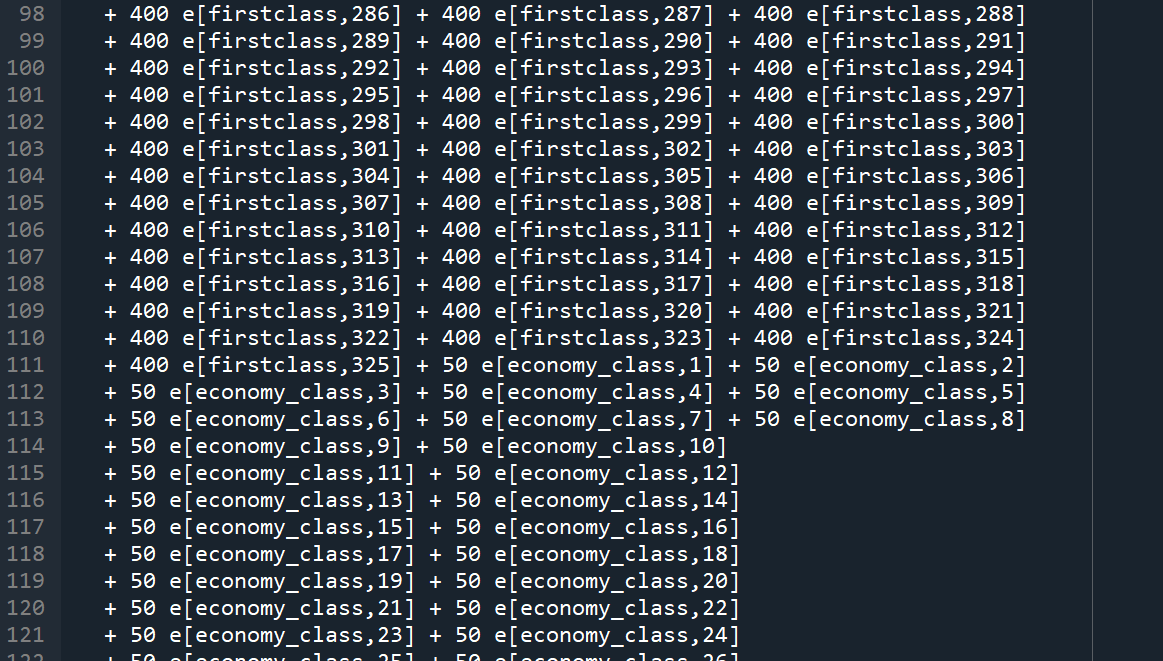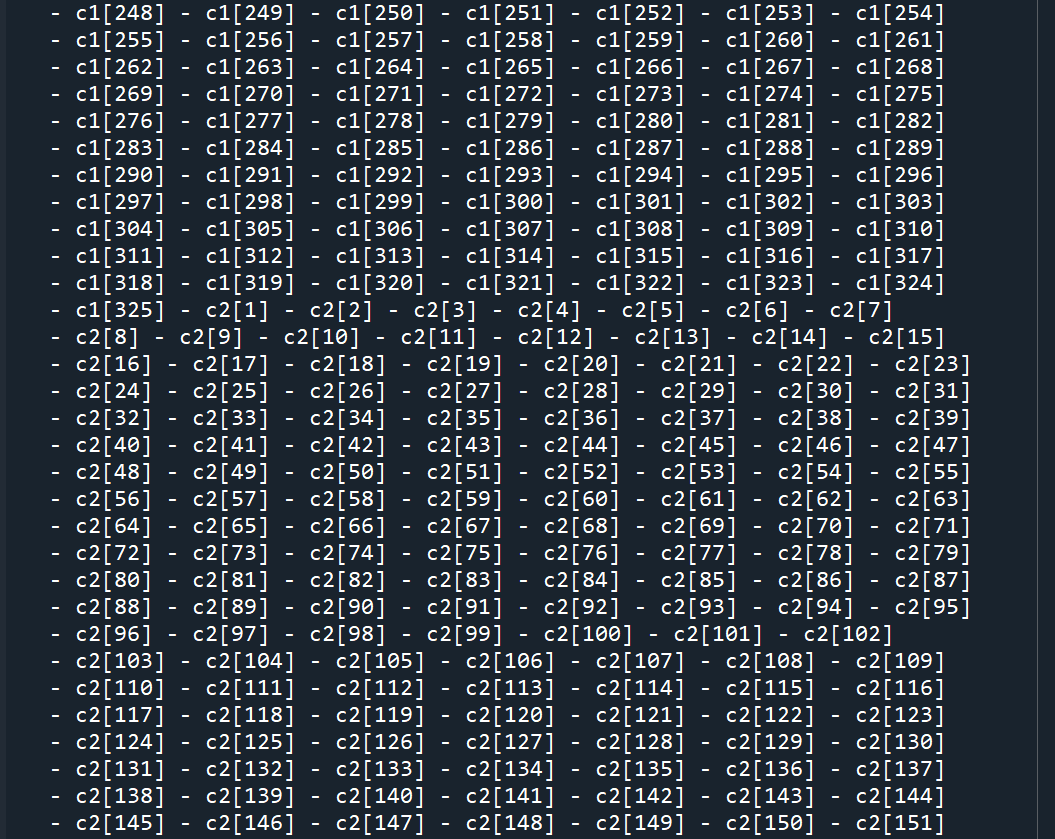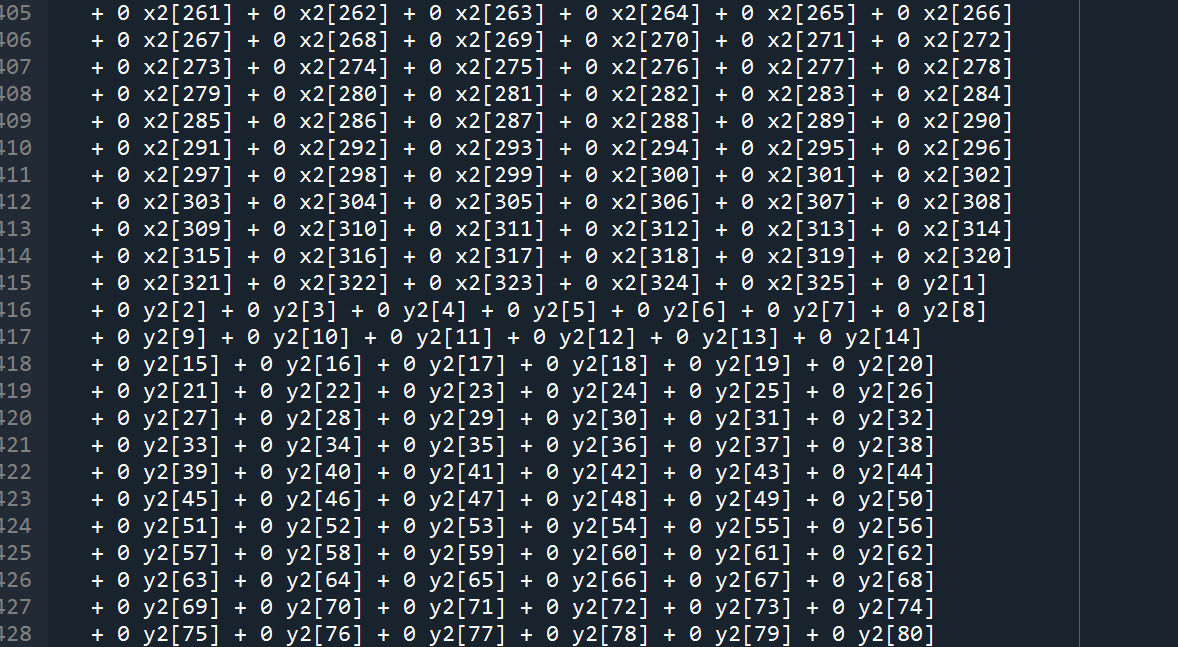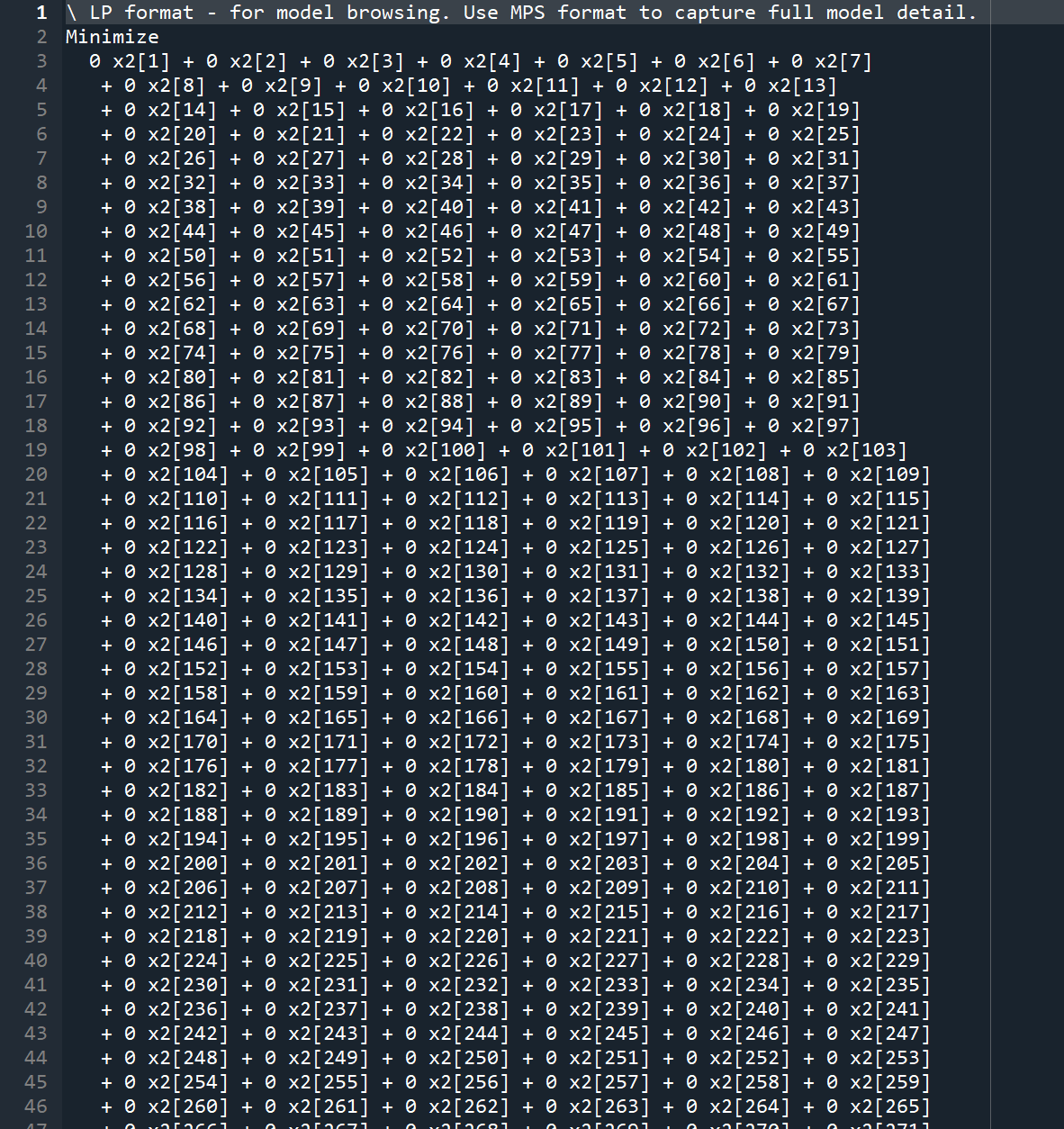•It seems like whether I set the objective or not, the output is the same.

•Community ModeratorHi Jingyue,

could you please share a minimal reproducible example of the code which produces such an outcome?
No need to attach any data, some hard-coded inputs will do.

Best regards
Jonasz

•Hi Jonasz,

the code regarding the objective function:

for h in patype:
for f in flights:
ehf[h,f] = 0

for f in flights:

cost1[f] = 0
cost2[f] = 0

patype, lh, rh = multidict({'firstclass':[100,500], 'economy class': [50,100]})

try:
m = Model()
print('building model')

x = m.addVars(tof.keys(), lb = 0, ub=287, vtype=GRB.CONTINUOUS, name='x')
# 变量tof_{ft}
e = m.addVars(ehf.keys(), obj = ehf, lb = 5, ub = 356, vtype=GRB.INTEGER, name='e')  # 变量n_{it}
c1 = m.addVars(cost1.keys(), vtype = GRB.CONTINUOUS, name = 'c1')
c2 = m.addVars(cost2.keys(), obj = cost2, vtype = GRB.CONTINUOUS, name = 'c2')

m.setObjective(quicksum(e.sum(h,'*') * (rh[h]-lh[h]) for h in patype)
- beta *(c1.sum('*') + c2.sum('*')), GRB.MAXIMIZE)

the output:

Coefficient statistics:
Matrix range     [1e+00, 5e+02]
Objective range  [1e+00, 1e+00]
Bounds range     [1e+00, 5e+02]
RHS range        [2e-01, 5e+02]
Presolve removed 211289 rows and 388741 columns
Presolve time: 2.05s
Presolved: 5200 rows, 8299 columns, 180222 nonzeros
Variable types: 5847 continuous, 2452 integer (2125 binary)

Root relaxation: objective 5.800000e+01, 2207 iterations, 0.03 seconds

Nodes    |    Current Node    |     Objective Bounds      |     Work
Expl Unexpl |  Obj  Depth IntInf | Incumbent    BestBd   Gap | It/Node Time

*    0     0               0      58.0000000   58.00000  0.00%     -    2s

Explored 0 nodes (2493 simplex iterations) in 2.30 seconds
Thread count was 8 (of 8 available processors)

Solution count 1: 58

Optimal solution found (tolerance 1.00e-04)
Best objective 5.800000000000e+01, best bound 5.800000000000e+01, gap 0.0000%

However the results are the same whether I set the objective or remove the objective from the model.

I'm really confused.

•Hi,

I've tried to write the model in lp format, and here are some screenshots of the lp document:

when I write the objective function as:

m.setObjective(quicksum(e.sum(h,'*') * (rh[h]-lh[h]) for h in patype)
- beta *(c1.sum('*') + c2.sum('*')), GRB.MAXIMIZE)

screenshots:when removing the objective function: the lp documentStill the output 'best objective' is not changed with or without the objective function.

I'm wondering if this has anything to do with the x2 and y2.

x2 and y2 are general constraint indicators, and the constraints states like this:

for f in tof.keys():

m.addConstr(quicksum(i* ui[i] for i in range(500)) == t.sum('*',f), 'index1')

m.addConstr(t.sum('*',f) >= x[f] - M * (1-s[f]), 'BigM1-1')
m.addConstr(t.sum('*',f) <= x[f] + M * s[f], 'BigM1-2')

m.addConstr(x[f] + 6 >= t.sum('*',f) - M * (1-hh[f]), 'BigM2-1')
m.addConstr(x[f] + 6 <= t.sum('*',f) + M * hh[f], 'BigM2-2')

m.addConstr(z[f] >= t.sum('*',f) - M * (1-h2[f]), 'BigM3-1')
m.addConstr(z[f] <= t.sum('*',f) + M * h2[f], 'BigM3-2')

for f in tof.keys():
m.addGenConstrIndicator(x2[f], True, y.sum('*', '*'), GRB.LESS_EQUAL, qk, 'c2')
m.addGenConstrIndicator(y2[f], True, y.sum('*', p), GRB.LESS_EQUAL, xp, 'c8')

•Gurobi Staff

Please note that the code you posted is not reproducible, because the flights list is missing. Additionally, patype is used before it is defined.

•Hi Jaromil,

I've tried again and everytime the objective value is the same, whether I set objective function or not.

No matter how objective funtion changes, the results are the same.

flights =  ['1', '2', '3', '4', '5', '6', '7', '8', '9', '10', '11', '12', '13', '14', '15', '16', '17', '18', '19', '20', '21', '22', '23', '24', '25', '26', '27', '28', '29', '30']

Deptime_base = {'1': 160.0, '2': 262.0, '3': 176.0, '4': 209.0, '5': 196.0, '6': 86.0, '7': 228.0, '8': 195.0, '9': 97.0, '10': 144.0, '11': 117.0, '12': 229.0, '13': 196.0, '14': 209.0, '15': 175.0, '16': 160.0, '17': 86.0, '18': 68.0, '19': 131.0, '20': 259.0, '21': 90.0, '22': 136.0, '23': 226.0, '24': 136.0, '25': 169.0, '26': 190.0, '27': 177.0, '28': 174.0, '29': 194.0, '30': 130.0}

Arrtime_base ={'1': 186.0, '2': 278.0, '3': 199.0, '4': 228.0, '5': 218.0, '6': 115.0, '7': 249.0, '8': 220.0, '9': 119.0, '10': 162.0, '11': 136.0, '12': 246.0, '13': 214.0, '14': 231.0, '15': 200.0, '16': 177.0, '17': 114.0, '18': 91.0, '19': 143.0, '20': 275.0, '21': 118.0, '22': 152.0, '23': 249.0, '24': 162.0, '25': 241.0, '26': 216.0, '27': 201.0, '28': 198.0, '29': 213.0, '30': 205.0}

patype, lh, rh = multidict({'firstclass':[100,500], 'economy class': [50,100]})

Findex = {}

for f in flights:

Findex[f] = 1

x = m.addVars(Findex.keys(), lb = 0, ub=287, vtype=GRB.CONTINUOUS, name='x')

z = m.addVars(Findex.keys(), lb = 0, ub = 500, vtype=GRB.CONTINUOUS, name='z')

beta = 1

The objective funtion goes like this:

m.setObjective(quicksum((e[h,f] * (rh[h]-lh[h]) for h in patype for f in Findex.keys()))
- beta * sum((c1[f] + c2[f] for f in Findex.keys())), GRB.MAXIMIZE)

c1[f] and c2[f] represents absolute value terms of the objective, and the constraints for them are:

x[f] - Deptime_base[f], 't1')
Deptime_base[f] - x[f], 't2')
z[f] - Arrtime_base[f], 't1-1')
Arrtime_base[f] - z[f], 't2-2')

I'm so confused and I would really appreciate it if you could answer this question!!!

•Gurobi Staff

Can you please post one complete minimal reproducible example? In your latest post, you are using $$\texttt{e}$$ and $$\texttt{rh}$$, which you defined in your previous post. This is very confusing. Thus, can you please edit your last post such that the code is self-contained and reproducible, i.e., one can copy and paste it and it will still work?

•Sure, no problem, I'm sorry for the confusion.

from gurobipy import *
flights = ['1','2','3','4','5','6','7','8','9','10','11','12','13','14','15','16','17','18','19','20','21','22','23','24','25','26','27','28','29','30']
Deptime_base = {'1': 160.0, '2': 262.0, '3': 176.0, '4': 209.0, '5': 196.0, '6': 86.0, '7': 228.0, '8': 195.0, '9': 97.0, '10': 144.0, '11': 117.0, '12': 229.0, '13': 196.0, '14': 209.0, '15': 175.0, '16': 160.0, '17': 86.0, '18': 68.0, '19': 131.0, '20': 259.0, '21': 90.0, '22': 136.0, '23': 226.0, '24': 136.0, '25': 169.0, '26': 190.0, '27': 177.0, '28': 174.0, '29': 194.0, '30': 130.0}

Arrtime_base ={'1': 186.0, '2': 278.0, '3': 199.0, '4': 228.0, '5': 218.0, '6': 115.0, '7': 249.0, '8': 220.0, '9': 119.0, '10': 162.0, '11': 136.0, '12': 246.0, '13': 214.0, '14': 231.0, '15': 200.0, '16': 177.0, '17': 114.0, '18': 91.0, '19': 143.0, '20': 275.0, '21': 118.0, '22': 152.0, '23': 249.0, '24': 162.0, '25': 241.0, '26': 216.0, '27': 201.0, '28': 198.0, '29': 213.0, '30': 205.0}

patype, lh, rh = multidict({'firstclass':[100,500], 'economy class': [50,100]})
beta = 1

Findex = {}
ehf = {}

for f in flights:
Findex[f] = 1

for h in patype:
for f in flights:
ehf[h,f] = 1
m = Model()

x = m.addVars(Findex.keys(), lb = 0, ub=287, vtype=GRB.CONTINUOUS, name='x')

z = m.addVars(Findex.keys(), lb = 0, ub = 500, vtype=GRB.CONTINUOUS, name='z')

e = m.addVars(ehf.keys(), lb = 5, ub = 356, vtype=GRB.INTEGER, name='e')

c1 = m.addVars(Findex.keys(), vtype = GRB.CONTINUOUS, name = 'c1')
c2 = m.addVars(Findex.keys(), vtype = GRB.CONTINUOUS, name = 'c2')

m.setObjective(sum(e[h,f]*(rh[h]-lh[h]) for h in patype for f in Findex.keys()) - beta * sum(c1[f] + c2[f] for f in Findex.keys()), GRB.MAXIMIZE)
# m.setObjective(sum(e[h,f]*(rh[h]-lh[h]) for h in patype for f in Findex.keys()), GRB.MAXIMIZE)
# m.setObjective(beta * sum(c1[f] + c2[f] for f in Findex.keys()), GRB.MINIMIZE)

m.addConstr(c1[f] >= x[f] - Deptime_base[f], 't1')
m.addConstr(c1[f] >= Deptime_base[f] - x[f], 't2')
m.addConstr(c2[f] >= z[f] - Arrtime_base[f], 't1-1')
m.addConstr(c2[f] >= Arrtime_base[f] - z[f], 't2-2')

for a in range(30):

for h,f in list(ehf.keys()):

if h == list(ehf.keys())[a]:

for a in range(30,60):
for h,f in list(ehf.keys()):
if h == list(ehf.keys())[a]:

m.optimize()
m.write('minormodel.lp'）

It seems that the minimal example works well........

•However, there are other constraints affecting the x[f] and z[f], should I add them to the minimal example and try?

•Gurobi Staff

Thank you for the example. It works perfectly.

Optimizing with the objective function

m.setObjective(sum(e[h,f]*(rh[h]-lh[h]) for h in patype for f in Findex.keys()) - beta * sum(c1[f] + c2[f] for f in Findex.keys()), GRB.MAXIMIZE)

and having a look at the solution point

m.optimize()m.write("solution.sol")

you can see that all $$\texttt{c}$$ variables have value $$0$$ at the optimal solution point. Thus, removing the

- beta * sum(c1[f] + c2[f] for f in Findex.keys())

part from the objective does not have any effect on the objective value.

Additionally, please note that the model is solved during presolve, i.e., Gurobi is able to prove the optimality of the solution without going into the branch-and-bound algorithm. Your model has only 180 variables but over 162k constraints. I suppose that all those constraints limit the solution space so much that an optimal solution value can be proven through presolving routines only. Note that when you switch to

m.setObjective(sum(e[h,f]*(rh[h]-lh[h]) for h in patype for f in Findex.keys()) - beta * sum(c1[f] + c2[f] for f in Findex.keys()), GRB.MINIMIZE)

the model becomes unbounded. Thus, the objective value has indeed an impact. It is only very limited due to the thousands of constraints compared to only 180 variables.

Does this help?

However, there are other constraints affecting the x[f] and z[f], should I add them to the minimal example and try?

If they are required to show the issue then yes please.

•Hi Jaromil,

Thanks for your advice!! I've tried to add all the constraints for a minor example with 20 data and the objective outputs are with no problem. However, when I use the whole data set, the model is infeasible thus I computing the IIS and feasRelaxS, and then the results are all the same whether I tried with the whole objective fuction (1) or some part (2) or (3):

m.setObjective(sum(e[h,f]*(rh[h]-lh[h]) for h in patype for f in Findex.keys()) - beta * sum(c1[f] + c2[f] for f in Findex.keys()), GRB.MAXIMIZE)   (1)
m.setObjective(sum(e[h,f]*(rh[h]-lh[h]) for h in patype for f in Findex.keys()), GRB.MAXIMIZE)   (2)
m.setObjective(beta * sum(c1[f] + c2[f] for f in Findex.keys()), GRB.MINIMIZE)   (3)

The output:

Gurobi Optimizer version 9.1.2 build v9.1.2rc0 (win64)
Thread count: 4 physical cores, 8 logical processors, using up to 8 threads
Optimize a model with 26003 rows, 168525 columns and 1334300 nonzeros
Model fingerprint: 0x7bb9aec3
Model has 5850 general constraints
Variable types: 1950 continuous, 166575 integer (165925 binary)
Coefficient statistics:
Matrix range     [1e+00, 1e+03]
Objective range  [5e+01, 4e+02]
Bounds range     [1e+00, 5e+02]
RHS range        [2e-01, 2e+03]
Presolve removed 2503 rows and 976 columns
Presolve time: 0.06s

Explored 0 nodes (0 simplex iterations) in 0.09 seconds
Thread count was 1 (of 8 available processors)

Solution count 0
No other solutions better than -1e+100

Model is infeasible
Best objective -, best bound -, gap -
Warning: to let Gurobi read it back, use rlp format
The model is infeasible; computing IIS

IIS computed: 1 constraints, 2 bounds
IIS runtime: 0.00 seconds
IIS is minimal

The following constraint(s) cannot be satisfied:
auxiliary1
model optimization
The model is infeasible; relaxing the constraints
Changed value of parameter timeLimit to 1000.0
Prev: inf  Min: 0.0  Max: inf  Default: inf
Gurobi Optimizer version 9.1.2 build v9.1.2rc0 (win64)
Thread count: 4 physical cores, 8 logical processors, using up to 8 threads
Optimize a model with 26003 rows, 196154 columns and 1361929 nonzeros
Model fingerprint: 0x7ce1a455
Model has 5850 general constraints
Variable types: 29579 continuous, 166575 integer (165925 binary)
Coefficient statistics:
Matrix range     [1e+00, 1e+03]
Objective range  [1e+00, 1e+00]
Bounds range     [1e+00, 5e+02]
RHS range        [2e-01, 2e+03]
Presolve removed 24376 rows and 190449 columns (presolve time = 8s) ...
Presolve removed 19828 rows and 187855 columns
Presolve time: 9.10s
Presolved: 6175 rows, 8299 columns, 182822 nonzeros
Variable types: 5844 continuous, 2455 integer (2126 binary)
Found heuristic solution: objective 63305.200000

Root simplex log...

Iteration    Objective       Primal Inf.    Dual Inf.      Time
0    5.8000000e+01   7.819900e+03   0.000000e+00      9s
2236    5.8000000e+01   0.000000e+00   0.000000e+00      9s

Root relaxation: objective 5.800000e+01, 2236 iterations, 0.05 seconds

Nodes    |    Current Node    |     Objective Bounds      |     Work
Expl Unexpl |  Obj  Depth IntInf | Incumbent    BestBd   Gap | It/Node Time

0     0   58.00000    0  261 63305.2000   58.00000   100%     -    9s
H    0     0                    12655.200000   58.00000   100%     -    9s
H    0     0                    12616.200000   58.00000   100%     -    9s
H    0     0                    12524.800000   58.00000   100%     -    9s
H    0     0                    12479.200000   58.00000   100%     -    9s
0     0   58.00000    0  612 12479.2000   58.00000   100%     -    9s
H    0     0                    1026.8000000   58.00000  94.4%     -    9s
0     0   58.00000    0  581 1026.80000   58.00000  94.4%     -    9s
*    0     0               0      58.0000000   58.00000  0.00%     -   10s

Cutting planes:
Gomory: 39
MIR: 616
Relax-and-lift: 11

Explored 1 nodes (3956 simplex iterations) in 10.40 seconds
Thread count was 8 (of 8 available processors)

Solution count 7: 58 1026.8 12479.2 ... 63305.2

Optimal solution found (tolerance 1.00e-04)
Best objective 5.800000000000e+01, best bound 5.800000000000e+01, gap 0.0000%

Slack values:
ArtP_auxiliary1 = 47.6
ArtP_auxiliary1 = 10.4
Over.

I'm wondering what is affecting the results and how to solve this problem.

•Gurobi Staff

Hi Jingyue,

Did you try writing the IIS to a file? Luckily your IIS is very small

IIS computed: 1 constraints, 2 boundsIIS runtime: 0.00 secondsIIS is minimal

Thus, you should have no problems finding out why your model is infeasible.

You can write the IIS to a file via

model.computeIIS()model.write("myIIS.ilp")

You should then inspect the $$\texttt{myIIS.ilp}$$ file to understand why your model is infeasible.